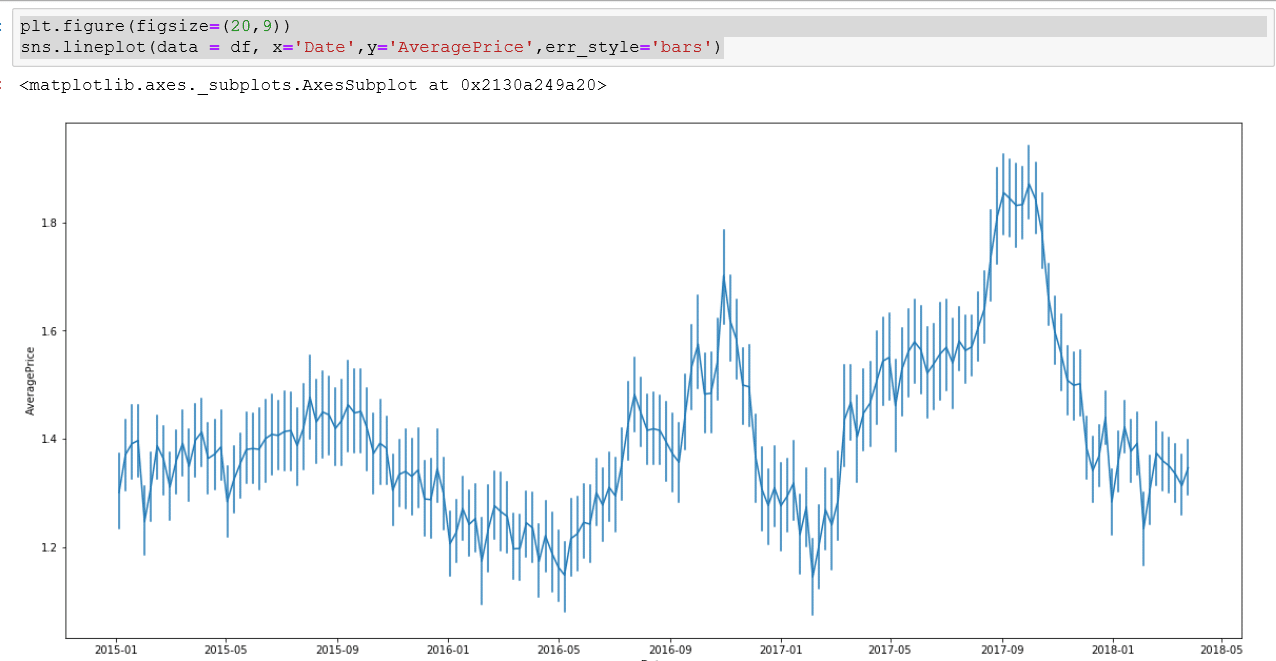# Seaborn Line Chart

Line graphs don’t have to be boring.  Seaborn line charts add a whole new opportunity to make your line charts stand out and communicate new insights.

Follow these easy to follow tutorial on how to to create line chart in Seaborn. There specifics that will be covered are colors, style and error bars.

The  fist step is to load the necessary libraries that. For this example we will be using Pandas to bring the data, Matplotlib for styling and Seaborn for creating graphs.

`#Load in the librariesimport pandas as pdimport numpy as npimport matplotlib.pyplot as pltimport seaborn as sns%matplotlib inline`

Now that you have your libraries, you can load in the data set. For this tutorial, we will be using  avocado dataset.  You can get the data set here.

`# Load in the datadf = pd.read_csv('avocado.csv')'#check the headdf.head()`

For this exaple, its important that we have a date in the right format.  You can check the format. In this data set the “Date” column is set at as an object. We want to convert this to datetime format.

If you already have your data in datetime format, you can skip this step.

`df['Date'] = pd.to_datetime(df.Date)`

Now that the data is in the right format. Lets use the Seaborn lineplot() function to procduce our initial line plot.  For the bare minimum of this function you need the x-axis,y-axis and actual data set.

`# This will create a line plot of price over timesns.lineplot(data=df, x='Date',y='AveragePrice')`

This is kind of bunched up. So I am going incrase the size of the plot by using:

`plt.figure(figsize=(20,9) sns.lineplot(data=df, x='Date',y='AveragePrice')`

Your linechart has error bands by default. You have the ability to turn these into bars or turn them off using the additional parameter.  The parameter

err_style =’bars’

This will change your error bands to bars.

`plt.figure(figsize=(20,9))sns.lineplot(data = df, x='Date',y='AveragePrice',err_style='bars')`

Create Multiple line plots with HUE:

We can add multiple line plots by using the hue parameter.  You can create multiple lines by grouping variables. In the avocado data set, we have organic and convential avocados in the column type. We can plot these by using the hue parameter.

`plt.figure(figsize=(20,9))sns.lineplot(data = df, x='Date',y='AveragePrice',err_style='bars', hue='type')You can find many other way to style this Seaborn lineplot by visiting the Seaborn website`

• in
• |
• August 13, 2019#### Gaelim Holland

Subscribe
Notify of